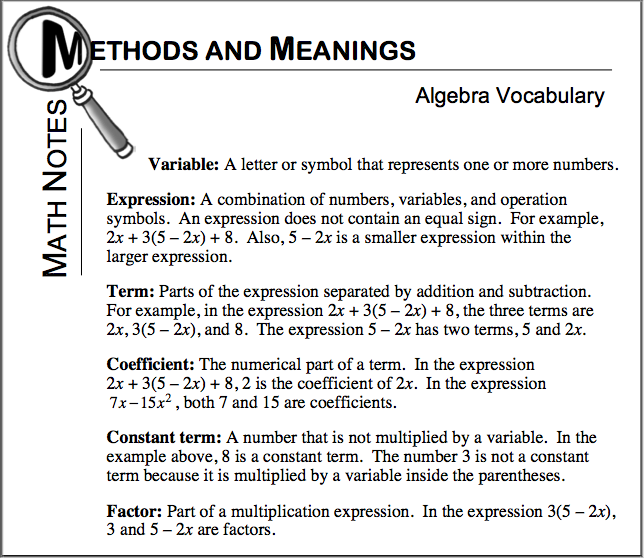### Home > CC2MN > Chapter 6 Unit 6B > Lesson CC2: 6.1.3 > Problem6-31

6-31.

MATH TALK

Read the Math Notes box in this lesson to review commonly used algebra vocabulary. Then consider the expression below as you answer the following questions. Homework Help ✎

$3x^2+7-2(4x+1)$1. Name a constant.

$7$

2. What are the two factors in $2(4x+1)$? What are the two factors in $4x$?

$2$ and $4x+1$; $4$ and $x$

3. Write an expression with a variable $m$, a coefficient $−3$, and a constant of $17$.

4. Use the words coefficient, constant, term, expression, variable, and factor to describe $4x^2+11y-37$.

Refer to the Math Notes box above.

5. Use the words factor, product, quotient, and sum to describe the parts of $\frac { 5 - m } { n } - 2 - 8 ( m + n )$.